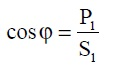What is power factor and current? Power triangle

# What is power factor and current? Power triangle

## What is the power factor and power triangle?

In the modern electrical distribution system, most of the loads are inductive in nature like motor,transformer, electrical furnace.

when induction motor run on no load or light load, create lagging power factor.

Reactive power is important because inductive loads required a magnetic field for functioning.

Inductive loads use two types of power one is active power (KW) which is required to do useful work, for example, convert electrical energy into heat, light, motor output, etc.

Other power is reactive power(kVAR) require for creating a magnetic field.

Active power consumes watts and can be measured on a wattmeter. It is expressed in kilowatts (kW).

Reactive power doesn’t contribute in useful “work,” but circulates between the generator and the load in the transmission line.

Reactive power places a load on the power source, as well as on the power source’s distribution system.

Reactive power is read in kilo volt-amperes reactive (kVAR) .

Power factor is a ratio of active power P and apparent power S.power factor formula
A high power factor signals efficient utilization of electrical energy, while a low power factor indicates poor utilization of electrical energy.

To determine the power factor (PF), divide working power (kW) by apparent power (kVA).

In a linear or sinusoidal system, the result is also referred to as the cosθ i.e power factor.power factor

### Power triangle :Power triangle
Power triangle is often used to demonstrate the relation between KW, kVAR, and KVA.

Low power factor indicates you are not fully utilizing electrical power which you pay for.

## What is an electrical current

So let understand what is current and how it flows through the conductor.
Electrical current is a flow of electrons from negative to positive terminals.

Every electrical conductor has free electrons in it. All free electrons are in random motion because absent of electrical potential and moving randomly throughout the conductor body.

If numbers of free electrons are more, the material becomes a good conductor of electricity.Flow of current in conductor
When potential difference or voltage applied across the conductor terminal all negatively charged electrons start to move from the negative terminal to positive terminal and flow of electricity begin.

The flow of electrons in a definite path through the conductor is refer as electric current.

Conventionally flow of current direction is always opposite to that of electrons flow.

Hence electric current start from positive electrode to negative electrode.

Current is flow because of potential difference if potential difference absent there is no flow of current observed.

During current flow, conductor gets heated due to conductor materials(internal resistance) internal opposition to the flow of electrons.

Unit of electrical current is Ampere.

### Ampere is defined as,

One ampere of current is flowing through conductor if one coulomb of change flows across the conductor in one second time.

The bad conductor of electricity does not have free electrons to share or performing flow of current.

### Resistance :

Electric current is a flow of electrons through a conducting material. When electrons flow through a conductor, they collide with each other and also atoms and ions present in a conductor. The property of conductor due to which it opposes to flow of current is called as resistance. it denoted by R, and unit of resistance is ohm